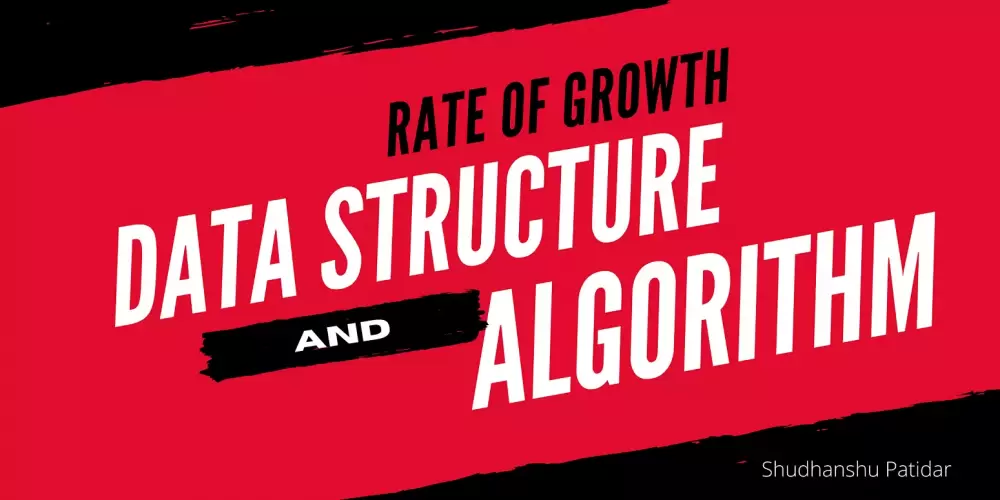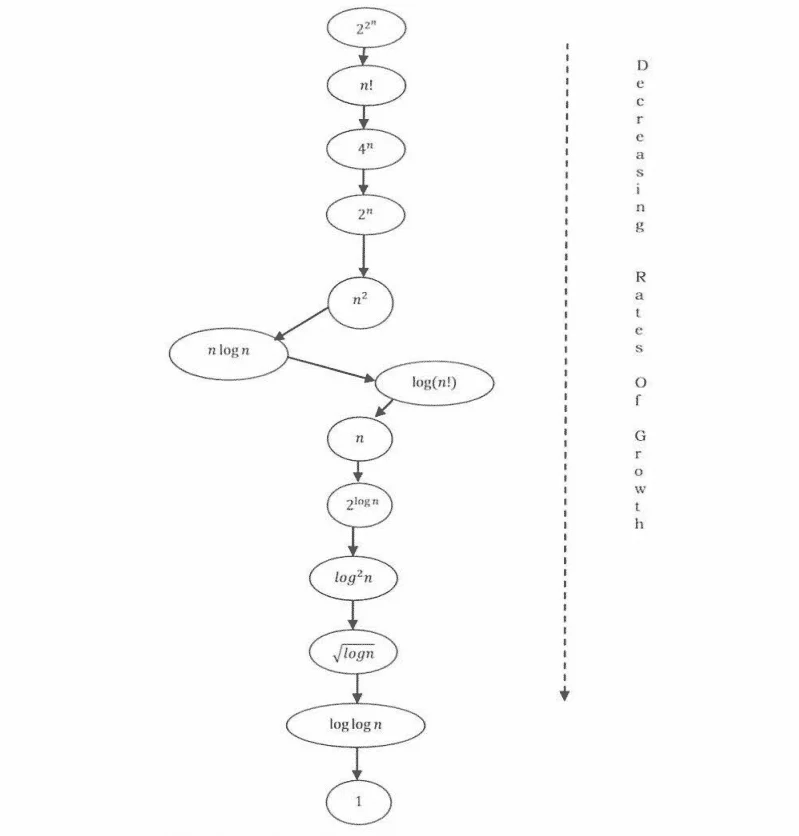## Rate of Growth in Data Structure and AlgorithmRate of Growth refers to the percentage change of a specific variable within a specific time period.

# What is the Rate of Growth?

The rate at which The running time increases as a function of input is called the rate of growth.

Let us assume that you go to a shop to buy a car and a bicycle. If your friend sees you there and asks what you are buying, then, in general, you say buying a car. This is because the cost of the car is high compared to the cost of the bicycle (approximating the cost of The bicycle to the cost of the car).

```  Total Cost = cost_of_car + cost_of _blcycle
Total Cost = cost_of _car (approximation)```

For the above-mentioned example, we can represent the cost of the car and the cost of the bicycle in terms of function, and for a given function ignore the low order terms that are relatively insignificant (for large value of input size, n). For example, in the case below, n4, 2n2, 100n, and 500 are the individual costs of some function and approximate to n4 since n4 is the highest rate of growth.

`n4 + 2n2 + 100n + 500 = n4`

# Commonly Used Rates of Growth

The diagram below shows the relationship between different rates of growth.Below is the list of growth rates you can be seen anywhere:

 Time Complexity Name Example 1 Constant Adding an Element to the front of the linked list. logn Logarithmic Finding an Element in sorted Array. n Linear Finding an Element in Unsorted Array. nlogn Linear Logarithmic Sorting n items by divide-and-conquer – Merge sort. n2 Quadratic The shortest path between two nodes in a graph. n3 Cubic Matrix Multiplication. 2n Exponential The Towers of Hanoi problem.